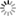##### Personal tools
You are here: Home Mathematics for AI

# Mathematics for AI

### - Overview

The relationship between Artificial Intelligence (AI) and mathematics can be summed up as: "A person working in the field of AI who doesn’t know math is like a politician who doesn’t know how to persuade. Both have an inescapable area to work upon!" Linear algebra, probability and calculus are the 'languages' in which machine learning is written. Learning these topics will provide a deeper understanding of the underlying algorithmic mechanics and allow development of new algorithms.

Probability and statistics are related areas of mathematics which concern themselves with analyzing the relative frequency of events. Probability deals with predicting the likelihood of future events, while statistics involves the analysis of the frequency of past events.

Linear algebra is a fundamental topic in the subject of mathematics and is extremely pervasive in the physical sciences. It also forms the backbone of many machine learning algorithms. Hence it is crucial for the deep learning practitioner to understand the core ideas.

### - Mathematics Behind Machine Learning

Many supervised machine learning and deep learning algorithms largely entail optimising a loss function by adjusting model parameters. To carry this out requires some notion of how the loss function changes as the parameters of the model are varied.

This immediately motivates calculus - the elementary topic in mathematics which describes changes of quantities with respect to another. In particular it requires the concept of a partial derivative, which specifies how the loss function is altered through individual changes in each parameter.

These partial derivatives are often grouped together - in matrices - to allow more straightforward calculation. Even the most elementary machine learning models such as linear regression are optimised with these linear algebra techniques.

A key topic in linear algebra is that of vector and matrix notation. Being able to 'read the language' of linear algebra will open up the ability to understand textbooks, web posts and research papers that contain more complex model descriptions. This will not only allow reproduction and verification of existing models, but will allow extensions and new developments that can subsequently be deployed in trading strategies.

Linear algebra provides the first steps into vectorisation, presenting a deeper way of thinking about parallelisation of certain operations. Algorithms written in standard 'for-loop' notation can be reformulated as matrix equations providing significant gains in computational efficiency.

Such methods are used in the major Python libraries such as NumPy, SciPy, Scikit-Learn, Pandas and Tensorflow. GPUs have been designed to carry out optimised linear algebra operations. The explosive growth in deep learning can partially be attributed to the highly parallelised nature of the underlying algorithms on commodity GPU hardware.

Linear algebra is a continuous mathematics subject but ultimately the entities discussed below are implemented in a discrete computational environment. These discrete representations of linear algebra entities can lead to issues of overflow and underflow, which represent the limits of effectively representing extremely large and small numbers computationally.

One mechanism for mitigating the effects of limited numerical presentation is to make use of matrix factorisation techniques. Such techniques allow certain matrices to be represented in terms of simpler, structured matrices that have useful computational properties.

Matrix decomposition techniques include Lower Upper (LU) decomposition, QR decomposition and Singular Value Decomposition (SVD). They are an intrinsic component of certain machine learning algorithms including Linear Least Squares and Pricipal Components Analysis (PCA). Matrix decomposition will be discussed at length later in this series.

It can not be overemphasised how fundamental linear algebra is to deep learning. For those that are aiming to deploy the most sophisticated quant models based on deep learning techniques—or are seeking employment at firms that are—it will be necessary to learn linear algebra extremely well.

[More to come ...]

##### Document Actions